Rational Expressions: More Simplifying (page 3 of 3)

Sections: Finding the domain, Simplifying rational expressions

• Simplify the following rational expression:
•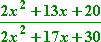Many students will try to do something like the following: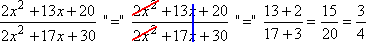Is this legitimate? Can the student really do this? (Think "bleeding, oozing...") You can NOT cancel term-by-term! You can ONLY cancel factors!

So the first thing I have to do (if I'm going to do the simplification correctly) is factor the numerator and the denominator: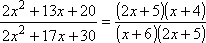Since the numerator and denominator share a common factor, I can reduce the expression as: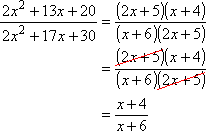Can I reduce any further? For instance, can I cancel off the x's? (whimpering, bleeding...) Can I cancel a 2 out of the 4 and the 6? (oozing, flopping...) No! This is as simplified as it's going to get, because there are no remaining common factors. Then the answer is: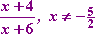Depending on your text, you might not need that "for x not equal to –5/2 part". However, since I cancelled off a "2x + 5" factor, this removed a division-by-zero problem from the original rational expression: 2x + 5 = 0 for x = –5/2.

• Simplify the following:
•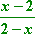The factors in the numerator and denominator are almost the same, but not quite, so they can't be cancelled — yet. If the fraction had been: Copyright © Elizabeth Stapel 2003-2011 All Rights Reserved

Advertisement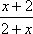...(that is, plusses instead of minuses), I could have rearranged the terms as: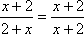...and cancelled to get "1", since order doesn't matter in addition. But order most-definitely does matter in subtraction, so I can't just flip the subtraction to get matching factors. However, take a look at this:

5 – 3 = 2
3 – 5 = –2

Do you see what happened? When I reversed the subtraction in the second line, I got the same number but the opposite sign. Then, if I flip a subtraction, I'll need to change the sign. So I can reverse one of the subtractions in the original rational expression above, as long as I remember to switch the sign out front: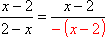Now I can cancel: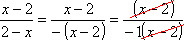Remember: If "nothing" is left, then a "1" is left, so: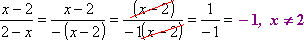(Depending on the text you're using, you may or may not need the "as long as x does not equal 2" part.)

You should keep this "flip a subtraction and kick a 'minus' sign out front" trick in mind. Depending on the text you're using, you may see a lot of this.

• Simplify the following expression:
•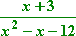To simplify this, I first need to factor. Then I can cancel off any common factors.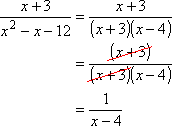Then the answer is: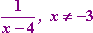(You might not need the "for all x not equal to –3" part.)

• Simplify the following expression:
•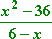To simplify this, I need to factor; I'll also need to flip the subtraction in the denominator, so I'll need to remember to change the sign.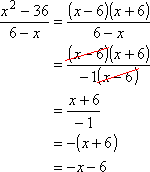Then the answer is: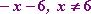(You might not need the "for all x not equal to 6" part.)

As you have probably noticed by now, simplifying rational expressions involves a lot of factoring. If you're feeling at all rusty on this topic, review now: simple factoring, factoring quadratics, and special factoring formulas.

<< Previous  Top  |  1 | 2 | 3  |  Return to Index

 Cite this article as: Stapel, Elizabeth. "Rational Expressions: More Simplifying." Purplemath. Available from     https://www.purplemath.com/modules/rtnldefs3.htm. Accessed [Date] [Month] 2016

MathHelp.com Courses
 Copyright © 2021  Elizabeth Stapel   |   About   |   Terms of Use   |   Linking   |   Site Licensing Contact Us